In :
using PyPlot
using NtToolBox
WARNING: Method definition macroexpand(Module, Any) in module Compat at C:\Users\Ayman\.julia\v0.5\Compat\src\Compat.jl:1491 overwritten in module MacroTools at C:\Users\Ayman\.julia\v0.5\MacroTools\src\utils.jl:64.

$\newcommand{\dotp}{\langle #1, #2 \rangle}$ $\newcommand{\umin}{\underset{#1}{\min}\;}$ $\newcommand{\RR}{\mathbb{R}}$ $\newcommand{\pd}{ \frac{ \partial #1}{\partial #2} }$ $\newcommand{\norm}{\|#1\|}$ $\newcommand{\pa}{\left(#1\right)}$

We consider the problem of finding a minimizer of a convex smooth function $\;f : \RR^d \rightarrow \RR$; that is, we want to solve $$\umin{x \in \RR^d} f(x)$$

Note that the minimizer is not necessarily unique.

The simplest method is gradient descent, which iteratively computes $$x^{(k+1)} = x^{(k)} - \tau \nabla f(x^{(k)}),$$ where $\nabla f(x) \in \RR^d$ is the gradient of $f$ at $x$, $x^{(0)} \in \RR^d$ is an arbitrary initial point, and the stepsize $\tau$ must satisfy $$0<\tau<2/\beta,$$ to have convergence, where $\beta$ is a Lipschitz constant of $\nabla f$; that is, $$\forall (x,x') \in \RR^N \times \RR^N, \quad \norm{\nabla f(x) - \nabla f(x')} \leq \beta \norm{x-x'}.$$

For instance, if $f$ is of class $C^2$, $$\beta= \sup_x \norm{Hf(x)},$$ where $Hf(x) \in \RR^{d \times d}$ is the Hessian of $f$ at $x$ and $\norm{\cdot}$ is the spectral operator norm (largest eigenvalue).

We consider a simple problem, corresponding to the minimization of a 2-D ($d=2$) quadratic form $$f(x) = \frac{1}{2} \pa{ x_1^2 + \eta x_2^2 } ,$$ where $\eta>0$ controls the anisotropy, and hence the difficulty, of the problem.

We can note that minimizing a strongly convex quadratic function is equivalent to solving a positive definite linear system. Gradient descent is then equivalent to the Richardson iteration applied to this linear system.

We define the anisotropy parameter $\eta$:

In :
eta = 8;

We define the function $f$:

In :
f = x -> (x^2 + eta*x^2) / 2;

Note: a 'lambda' function is a one-line function definition; in Matlab, this would be [email protected](x)(x(1)^2+eta*x(2)^2)/2;

An example of function evaluation:

In :
f([2,3])
Out:
38.0

We display the function using a contourplot:

In :
include("NtToolBox/src/ndgrid.jl")
t = linspace(-.7,.7,101)
(u, v) = meshgrid(t,t)
F = (u.^2 + eta.*v.^2) ./ 2
contourf(t, t, F, 35);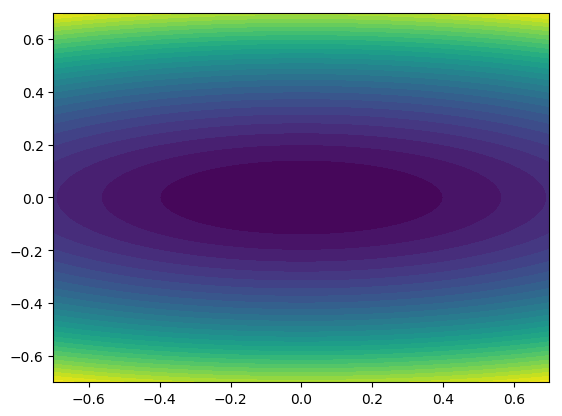We define the gradient of $f$:

In :
return[x; eta*x]
end;

An example of evaluation:

In :

Since $f$ is quadratic, its Hessian is the constant matrix $\left(\begin{array}{cc}1&0\\0&\eta\end{array}\right)$. Its spectral norm, which is the Lipschitz constant of Grad_f, is $\beta=\max(1,\eta)$.

The stepsize $\tau$ must satisfy $0< \tau < 2/\beta$.

In :
tau = 1.8/max(eta,1); tau
Out:
0.225

Now we implement the gradient descent method: given the initial estimate $x^{(0)}$ of the solution, the stepsize $\tau$ and the number $k$ of iterations, we compute $x^{(k)}$:

In :
nbiter = 10
x = [1, 1]  # initial estimate of the solution
for iter in 1:nbiter  # iter goes from 1 to nbiter
end
x   # to display x, like in Matlab. Use print(x) if this is not the last command of the cell, else nothing is displayed
Out:
2-element Array{Float64,1}:
0.0781658
0.107374
In :
f(x)
Out:
0.049171809817584886

We encapsulate the code in a function called GradientDescent.

In :
x = x0
for iter in 1:nbiter  # iter goes from 1 to nbiter
x = x - tau.*Grad_f(x)   # x has type 'array'. Like in C, one can also write x-=tau*Grad_f(x)
end
return x
end;
In :
Out:
2-element Array{Float64,1}:
0.0781658
0.107374

We define a function called GradDescentArray which puts the iterates in a 'matrix' (a 2-D array in fact); they are first put in a list and the list is converted to an array at the end (the + operation on lists concatenates them, whereas on arrays this is the classical elementwise addition).

In :
x = x0
xlist = [x0 x0]
for iter in 1:nbiter
xlist = [xlist; [x x]]
end
return xlist
end;
In :
Out:
11×2 Array{Float64,2}:
0.6         0.6
0.465      -0.48
0.360375    0.384
0.279291   -0.3072
0.21645     0.24576
0.167749   -0.196608
0.130005    0.157286
0.100754   -0.125829
0.0780845   0.100663
0.0605155  -0.0805306
0.0468995   0.0644245

We can access the elements of an array like in Matlab. Be careful: like in C, the first element has index 0! Some examples:

In :
xarray[2, 1]
Out:
0.46499999999999997
In :
xarray[2, :]
Out:
2-element Array{Float64,1}:
0.465
-0.48
In :
xarray[:, 1]
Out:
11-element Array{Float64,1}:
0.6
0.465
0.360375
0.279291
0.21645
0.167749
0.130005
0.100754
0.0780845
0.0605155
0.0468995

We can apply the function $f$ to every iterate $x^{(k)}$ of the list xarray, using the command map:

In :
mapslices(f, xarray, 2)
Out:
11×1 Array{Float64,2}:
1.62
1.02971
0.654759
0.416489
0.265017
0.168689
0.107407
0.0684076
0.043581
0.0277718
0.0177019

We plot the cost function $f(x^{(k)})$ as a function of $k$, in log-scale:

In :
plot(0:size(xarray) - 1, log10(mapslices(f, xarray, 2)), "o-");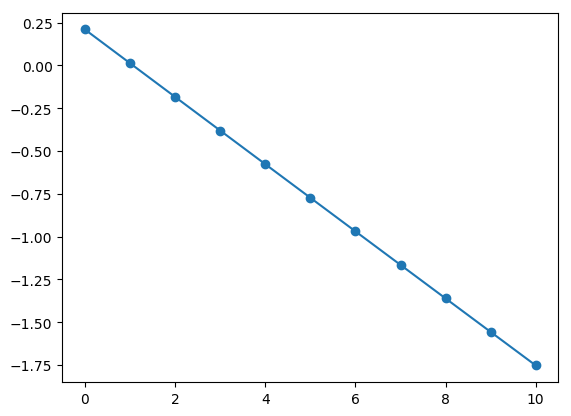We remark that because the function $f$ is strongly convex, the convergence is linear. Also, we can note that gradient descent is monotonic: $f(x^{(k)})$ is decreased at every iteration.

We plot the iterates above the contourplot of $f$:

In :
contourf(t, t, F, 35)
plot(xarray[:, 1], xarray[:, 2], "w.-")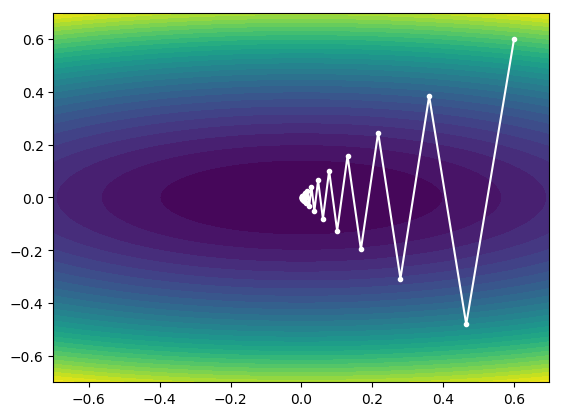Out:
1-element Array{Any,1}:
PyObject <matplotlib.lines.Line2D object at 0x000000000A23D828>

Gradient descent in large dimension: image restoration¶

Local differential operators like the discrete gradient are fundamental for variational image processing.

We load the image Lena in the array $x^\sharp$ (we choose the symbol # - 'sharp', because the image is clean, not degraded).

In :
name = "NtToolBox/src/data/lena.png"
println(size(xsharp))
println("The size of the image is $(size(xsharp,1)) x$(size(xsharp,2)).")
println("The range of the pixel values is [$(minimum(xsharp)),$(maximum(xsharp))].") # the range of the pixel values is [0.0, 1.0] because load_image scales the image.
(512,512)
The size of the image is 512 x 512.
The range of the pixel values is [0.0, 256.0].
In :
SystemError: opening file xsharp: No such file or directory

in #systemerror#51 at .\error.jl:34 [inlined]
in systemerror(::String, ::Bool) at .\error.jl:34
in open(::String, ::Bool, ::Bool, ::Bool, ::Bool, ::Bool) at .\iostream.jl:89
in #readdlm_auto#11(::Array{Any,1}, ::Function, ::String, ::Char, ::Type{T}, ::Char, ::Bool) at .\datafmt.jl:124
in #readdlm#7(::Array{Any,1}, ::Function, ::String, ::Char, ::Char) at .\datafmt.jl:73
in #readdlm#5(::Array{Any,1}, ::Function, ::String) at .\datafmt.jl:56

We display the image.

In :
imageplot(xsharp)
set_cmap("gray")
colorbar()       # displays the color bar close to the image
title("This is Lena");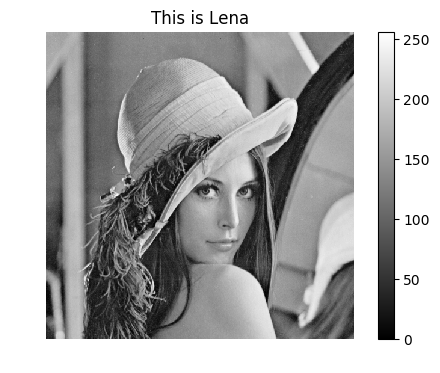We define the 'discrete gradient' $D$, which is the linear operator which maps an image to an image whose values are pairs of vertical and horizontal finite differences:

$$(D x)_{n_1,n_2} = \big((D x)_{n_1,n_2,v},(D x)_{n_1,n_2,h}\big)=( x_{n_1+1,n_2}-x_{n_1,n_2}, x_{n_1,n_2+1}-x_{n_1,n_2} ) \in \RR^2,$$

where $n_1$ and $n_2$ are the row and column numbers, respectively (the origin $n_1=n_2=0$ corresponds to the pixel at the top left corner) and where Neumann boundary conditions are assumed: a difference accross the boundary is zero.

Let us define the operator $D$ as a function:

In :
function D(x)
vdiff = [diff(x,1) ; zeros(1,size(x,2))] # concatenates along the rows
hdiff = [diff(x,2) zeros(size(x,1),1)]   # concatenates along the columns
return cat(3, vdiff, hdiff) # combination along a third dimension
end;
In :
v = D(xsharp);

We display the two components as two grayscale images.

In :
fig = figure(figsize=(16,7))
suptitle("The two images of vertical and horizontal finite differences")
subplot(1,2,1)
imageplot(v[:, :, 1])
title("Image of vertical finite differences")
set_cmap("gray")
colorbar()

subplot(1,2,2)
imageplot(v[:, :, 2])
colorbar()
title("Image of horizontal finite differences")
set_cmap("gray")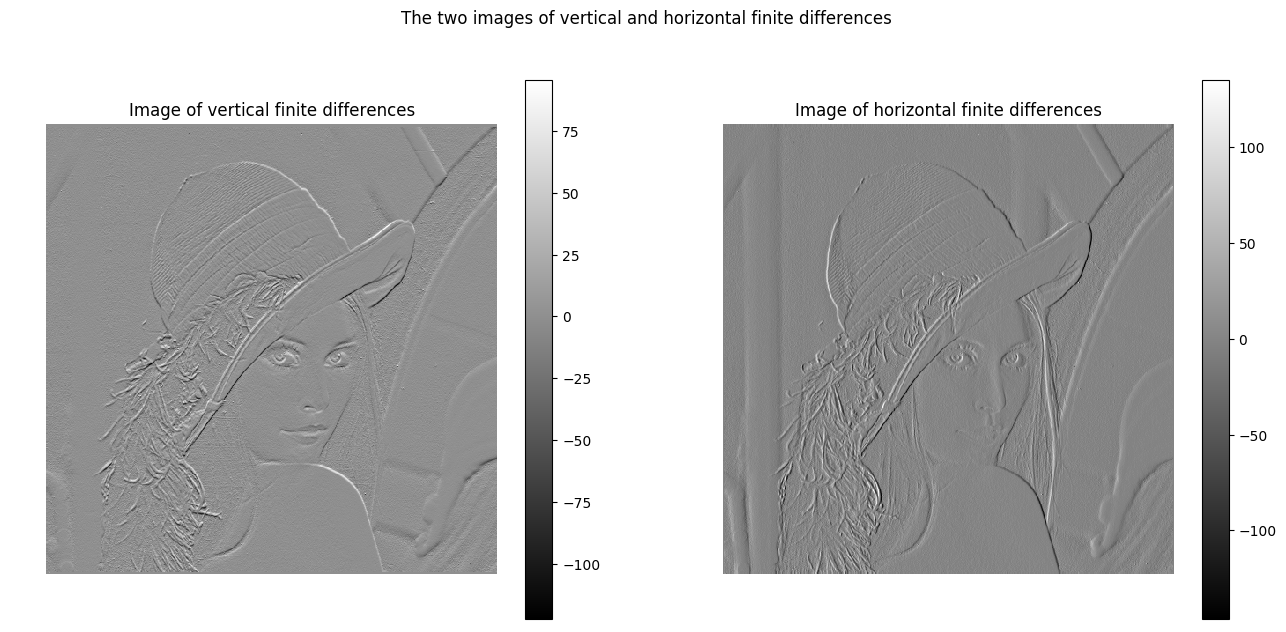Let us display the image of the magnitudes $\norm{(D x)_{n_1,n_2}}$, which are large near edges.

In :
imshow(sqrt(sum(v.^2, 3)[:, :]))
colorbar()